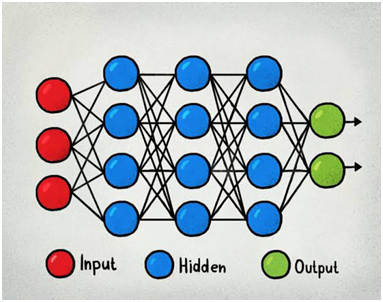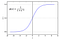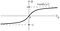# Importance of Activation Functions in Neural Networks

A neural network is a series of algorithms that works in a way the human brain does to establish relationship between a set of data. The human brain contains huge number of interconnected neurons which create a pathway for propagation of information through the brain. At first some neurons are triggered by an external stimulus, then those neurons trigger some other neurons and in this way the information is passed from one place to another.

The artificial neural network work in a similar way. Multiple layers with varying number of neurons are present and their interconnections create a complex network establishing relationship among all the neurons. Each neuron contains some data or information associated with a weight and a bias.A TYPICAL NEURAL NETWORK

The above is a neural network showing the input layer in red, the hidden layers in blue and the output layer in green. We see that there are 3 hidden layers in this particular neural network. However the number of hidden layers is a hyperparameter i.e. it depends on our need and we can change it accordingly. The number of neurons in each hidden layer is also a hyperparameter. Some data is fed to the input layer, the input undergoes a linear operation and then the activation function is applied to it in the hidden layers and the output is produced.

So what are activation functions?

Activation functions are extremely important for constructing a neural network. Activation functions are mathematical functions attached to each neuron. Application of the activation function tells us that which neurons in each layer will be triggered. Only the neurons with some relevant information are activated in every layer. The activation takes place depending on some rule or threshold. The main function of the activation function is to introduce non-linearity in the network.

But then the question comes that why do we even need non linearity?

X1, x2, x3,…,xn are the inputs to the neural network and w1, w2, w3,…,wn are the corresponding weights associated with the neurons. Without activation function the output after each layer is simply –

Y= Σ(xi*wi)+bias

This is a linear operation and this keeps getting repeated in every layer and the output is just a linear function of the input. Composition of several linear functions is also a linear function. So clearly all the hidden layers in between become useless and they collapse into one single layer performing a single linear operation. Thus the neural network becomes one layer deep. The neural network will be reduced to a simple linear regression model.

Although linear functions are easy to work with but their usage is very limited. They cannot be used to learn complex data such as image, audio, video etc.

However neural networks are expected to be able to perform much more complicated learning which linear regression could not. So if we don’t use activation function, the purpose of the neural networks will not be served. Most real life problems are complex and non linear so we need activation function for the network to solve them.

So now we can definitely understand why we need activation function so much.

Thus Y is the output after applying an activation function.

The output from the activation function moves to the next hidden layer and the same process is repeated. This forward movement of information is known as the forward propagation.

But what if the output generated is far away from the actual value? Using the output from the forward propagation, error is calculated. Based on this error value, the weights and biases of the neurons are updated. This process is known as back-propagation.

Non linear activation function is also extremely important because they are differentiable and make back-propagation(gradient descent) possible. Back-propagation minimizes the error and enhances the accuracy or efficiency of the model.

The most commonly non linear activation functions used are-

1. Sigmoid function:graph for Sigmoid function

Advantages-

· The output values are bound between 0 and 1. So the output of each neuron will be normalized.

· It provides a clear prediction. 0.5 is the threshold value for prediction.

Disadvantages-

· The function is not zero centered. Hence it is computationally complex.

· Vanishing gradient problem- When the values of X become drastically large or small, then the Y value becomes almost constant. Thus the gradient becomes very small and almost vanishes. This is the vanishing gradient problem. Consequently this problem affects back-propagation and slows down the learning process.

2. Tanh (Hyperbolic tangent)graph for tanh function

· It is bound to the range -1 to 1.

· It has a steeper slope than sigmoid function.

· But the vanishing gradient problem is here too. However this function is zero centered unlike sigmoid.

3. ReLu (Rectified Linear Unit)-

· It cheap to compute and accelerates the convergence of the gradient descent compared to the other activation functions.

· For the negative inputs the result is 0. The neuron does not get activated.

· Does not have the vanishing gradient problem.

· It is a much easier and efficient activation function than the other two.

## The Startup

Get smarter at building your thing. Join The Startup’s +724K followers.

## The Startup

Get smarter at building your thing. Follow to join The Startup’s +8 million monthly readers & +724K followers.## The Startup

Get smarter at building your thing. Follow to join The Startup’s +8 million monthly readers & +724K followers.

## More From Medium

Medium is an open platform where 170 million readers come to find insightful and dynamic thinking. Here, expert and undiscovered voices alike dive into the heart of any topic and bring new ideas to the surface. Learn more

Follow the writers, publications, and topics that matter to you, and you’ll see them on your homepage and in your inbox. Explore

If you have a story to tell, knowledge to share, or a perspective to offer — welcome home. It’s easy and free to post your thinking on any topic. Write on Medium